# Mean Radiant Temperature (MRT) calculation using Honeybee surface temp and Ladybug SolarCal

Hi everyone,

The papers “Wind, Sun, Surface Temperature, and Heat Island: Critical Variables for High-Resolution Outdoor Thermal Comfort” (Mackey et al., 2017) and “The Simulation of Mean Radiant Temperature in Outdoor Conditions: A review of Software Tools Capabilities” (Naboni et al., 2020) describe a method for the calculation of MRT.

I would like to use a similar method to evaluate the MRT at different assessment points (created using honeybee generate test points) between a simple block of four buildings (see figure below).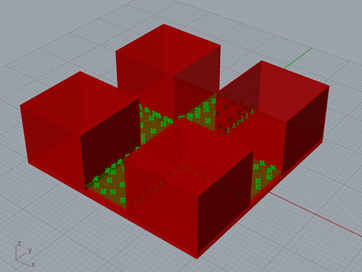However I have some questions regarding this calculation. As I understand, first you should calculate the surface temperatures of the buildings and ground using EnergyPlus. With these surface temperatures and the sky temperature, you can then calculate a base longwave MRT using the formulas below.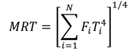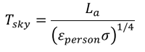I have now calculated the hourly surface temperatures for all surfaces. I was planning on calculating the view factors for the different surfaces (facades, ground, sky) as seen from each individual test point manually, and subsequently performing the MRT calculation in Python (see attached document). Is this the way to go? Or is there a component which performs the MRT calculation as described above?

Upon calculation of the base longwave MRT, the Ladybug Outdoor Solar Temperature Adjustor should then account for the shortwave radiation.

A final question, is there an example model using the calculation method as described in the papers? All examples that I’ve found so far seem to use the microclimate map component, which I believe is not the same?

Thanks in advance for helping!

test MRT LB HB.gh (607.5 KB)

Hi @n.dewaard ,

The general MRT-computing methods that you describe are correct and you certainly could draw the whole process out into several components like this example here. However, this workflow is so common that we have dedicated components for it, which will run much faster than the drawn out example. Here is a sample file showing the minimum that is needed to build a microclimate map:

The Longwave and shortwave MRT in that “Microclimate Map - Simple” example are computed exactly as you described.

Hi Chris,

Thanks a lot for your quick response. That clarifies a lot. I’ll have a look at the examples you’ve send me!

1 Like

hi @chris,

Thanks again for your response to my question. I’ve tried recreating the deconstructed microclimate map. However, I saw that this workflow is used to calculate MRT for an indoor situation. I’ve altered the workflow by adding a sphere, and giving this sphere a certain long-wave sky temperature Tsky. For the calculation of the long-wave based MRT, I then calculated the sky view factors (with the sphere) and multiplied these by the sky temperature. (see attached file)test MRT LB HB.gh (673.9 KB)

Now I do have a question about the calculation of the sky temperature. In the paper “Wind, Sun, Surface temperature, and Heat Island: Critical Variables for High-Resolution Outdoor Thermal Comfort”, the Tsky should be calculated according to the following formula: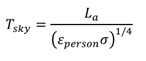However, when calculating Tsky this way, I get a very large value, which doesn’t make really make sense to me (see figure below). From several sources I’ve learned that the Tsky values should be within a range of -50 and +20 degrees Celsius (https://www.designingbuildings.co.uk/wiki/Sky_temperature#:~:text=The%20temperature%20in%20outer%20space,increasing%20the%20effective%20sky%20temperature.)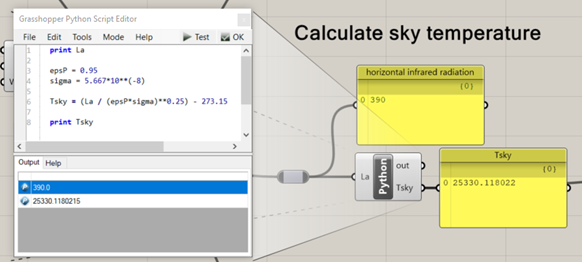From another source (“The Significance of Sky Temperature in the Assessment of the Thermal Performance of Buildings”), I’ve found the following formula to calculate Tsky:

Tsky = (horizontal_IR / sigma)^0.25 – 273.15

With this formula I get a much more sensible result (see figure below).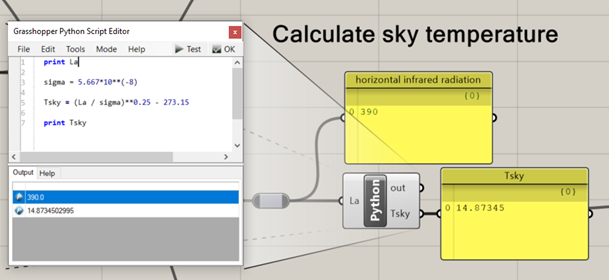Do you know whether I do something wrong when applying the first formula?
Thanks in advance for your help!

Sorry for the late reply, @n.dewaard ,

You can see the formula for sky temperature in the ladybug-core documentation:

You can see that we also use a default of 1 for the source emissivity there but, for the SolarCal model, we tend to use a default body emissivity of 0.95:

I think, with the 0.95 emissivity assumption, you will still find that you get sky temperatures between -50 and 20 C. But, yes, you will get some weird results if you set the emissivity to something low. The chances of that happening are pretty slim, though. I guess it you wrapped yourself in aluminum foil, you might be able to lower the emissivity and get some odd results.

Thanks for your help!

Also, I just re-read your original question and you are right that I misprinted the formula in the paper. Oh, man. I am sorry about that. So much for my editing skills and the peer review process. I am not sure that I can reach out to the IBPSA committee to change it or how much of the damage may already be done. I may just have to hope that my (much better) code documentation outlives my paper.

Here’s another software documentation source that gives the correct formula:

Actually, I know that I shouldn’t blame the peer review process here. I doubt that I would have ever caught an error like that in someone else’s paper that I was reviewing. I guess this just means that peer review isn’t over once the paper is published. And it also means that you are an excellent reviewer, @n.dewaard !

Thank you for catching the mistake.

I indeed found the correct formula in the ladybug code documentation. Thanks again for your response and sending me the links to the code documentation and other software documentation!

Hi @Chris and others,

As mentioned earlier, I’m working on a MSc thesis in which I will evaluate how PET-values outdoors change for different design parameters (think of H/W ratio streets, orientation streets etc.). One of the parameters of which I’d like to study the influence is surface albedo. Therefore it is important that in my MRT calculation, the reflection of direct radiation by façades is taken into account.

Thanks @Chris for implementing this in the new LBT plugin and thanks for pointing me at this method to convert values from Radiance into a shortwave MRT. I’ve imported the method into a GHPython component, and that seems to be working fine. However, I do have some questions regarding the Radiance input that goes into the component. I’ve tried doing some Radiance calculations (attached in the “01 testcase LBT and Radiance” file), for a simple geometry with one building in an open field (figure below), however since I don’t have any experience with Radiance, I’m not sure whether what I’m doing is correct.

My questions are all related to how to get the correct output from Radiance. The input parameters in the shortwave_from_horiz_components method are the following: diffuse horizontal solar irradiance, direct horizontal solar irradiance and ground-reflected horizontal irradiance. My two main questions are the following:

• All irradiance components needed are ‘horizontal irradiance’. How do I make sure to get these horizontal irradiance values from the Radiance calculation? When looking at direct radiation, the value you get from the epw file is ‘normal’ direct radiation. If I use this as input in the Radiance calculation (as I’ve done in my example file “01 testcase LBT and Radiance”) do I get ‘horizontal’ values out of the calculation?
• My second question is about splitting the ground reflected irradiance from the other irradiance values. I’ve tried to do this by subtracting and adding different radiation outcomes in the example file attached, however, I doubt whether it’s correct what I’ve done.

So to summarize my questions: I was wondering whether the ‘diffuse horizontal solar irradiance’, ‘direct horizontal solar irradiance’ and ‘ground-reflected horizontal irradiance’ I’ve used as input in my example file are correct. And if not, whether you might have any tips on how to calculate these values correctly from Radiance, or whether you might have an example file in which this is done.

I do think that something is still incorrect in the Radiance calculation in the example file, since when I compare it to the original method of calculating MRT using the SolarAdjustTemperature component from Ladybug Legacy, I get quite big differences for this particular testcase (see figures below and attached file with the legacy calculation). Especially at the edges of the ground area, there’s a clear difference. Here, out of reach of the influence of reflectivity of the building, the MRT should be approximately the same between the two methods if I’m correct.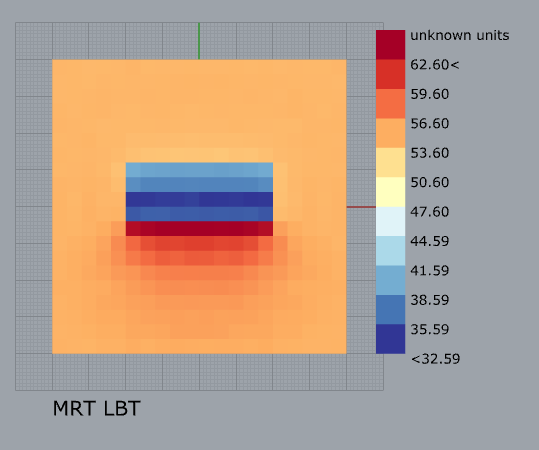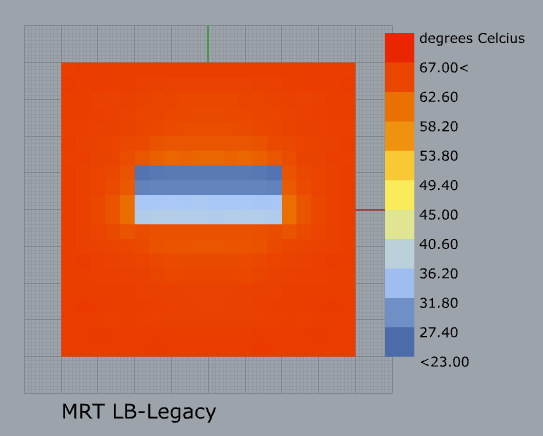Thanks again for helping me before, and any comments on improvement of this calculation would be greatly appreciated!

One final note: sometimes the Radiance calculations seem to be interfering with each other if I run them together. Running them separately, and sometimes multiple times solves this problem. I don’t know whether this has to do with the way I set up the Radiance calculation. Do more people experience these errors?

01 testcase Ladybug Legacy.gh (684.5 KB)

01 testcase LBT and Radiance.gh (765.9 KB)

One remark:
I’ve figured out that when you enter the direct normal radiation, the output of the Radiance calculation is indeed the direct horziontal radiation.

If I’m correct: when the entered direct normal irradiance is 563, and the zenith of this particular hour is 35 degrees, then the direct horziontal irradiance is indeed 462.75 according to the formula below:

DHI = cos(zenith)*DNI

However, I’m still puzzled about what causes the difference between MRT with the legacy method and LBT method as well as how to seperate the ground reflected irradiance from the overall irradiance. So I would still very much appreciate any help with these questions! Thanks in advance